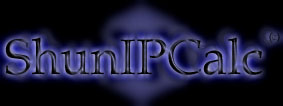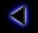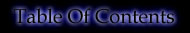.
Binary operators
.
The binary AND is defined as follows:

0 AND 0 = 0
1 AND 0 = 0
0 AND 1 = 0
1 AND 1 = 1

"1 if both bits to , 0 otherwise"

The binary OR is defined as follows:
0 OR 0 = 0
1 OR 0 = 1
0 OR 1 = 1
1 OR 1 = 1

"1 if one bit is 1 or both are 1, 0 otherwise"

The binary XOR (eXclusive OR) is defined as follows:
0 XOR 0 = 0
1 XOR 0 = 1
0 XOR 1 = 1
1 XOR 1 = 0

"1 if one bit is 1 or the other to 1 but not NOT both to 1"

The binary NOT (inversion) is defined as follows:
NOT 0 = 1
NOT 1 = 0

"1 if 0, 0 if 1" : invert the bit

.
..
Copyright (c) 2002 - Guillaume Péan
www.shunsoft.net